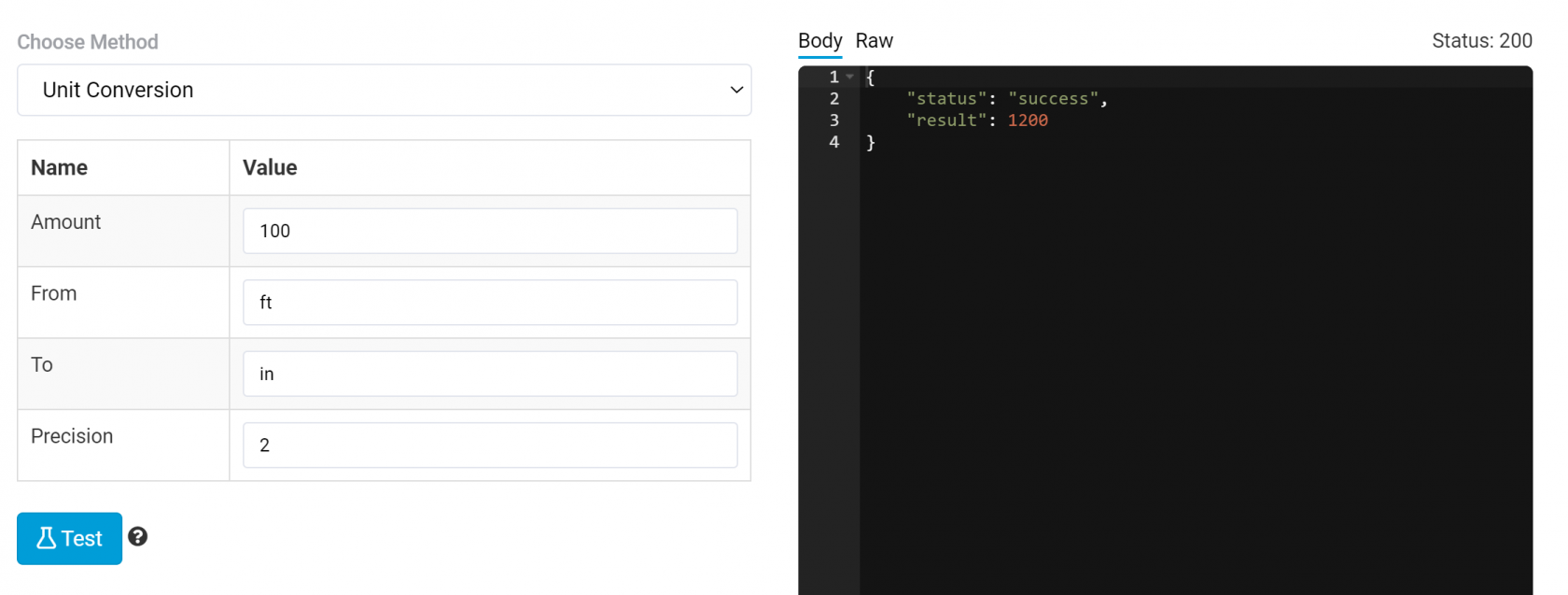# Unit Conversion

The unit conversion pipe enables you to convert numbers from one unit to another either while data is being loaded or when being saved.

To convert a number, you must pass the following 4 parameter values:

• Amount
• From
• To
• Precision

Amount is the numeric value to be converted.

From is where you're converting from. See list below of all available options.

To is what you'd like to convert it to.

Precision is how many decimal places should be used.Below is the list of available units.

Remember you can only convert within the same category. For example, you can convert from Length to Volume.

## Length

• m - Meter
• km - Kilometer
• dm - Decimeter
• cm - Centimeter
• mm - Milimeter
• μm - Micrometer
• nm - Nanometer
• pm - Picometer
• in - Inch
• ft - Foot
• yd - Yard
• mi - Mile
• h - Hand
• ly - LightYear
• au - Astronomical Unit
• pc - Parsec

## Area

• m2 - Square Meter
• km2 - Square Kilometer
• cm2 - Square Centimeter
• mm2 - Square Milimeter
• ft2 - Square Foot
• mi2 - Square Mile
• ac - Acre
• ha - hectare

## Volume

• l - Litre
• ml - Mililitre
• m3 - Cubic Meter
• pt - Pint
• gal - Galon

## Weight

• kg - Kilogram
• g - Gram
• mg - Miligram
• N - Newton (based on earth gravity)
• st - Stone
• lb - Pound
• oz - Ounce
• t - Metric Tonne
• ukt - UK Long Ton
• ust - US short Ton

## Speed

• mps - Meters per Second
• kph - Kilometers Per Hour
• mph - Miles Per Hour

## Rotation

• deg - Degrees

## Temperature

• k - Kelvin
• f - Fahrenheit

## Pressure

• pa - Pascal
• kpa - kilopascal
• mpa - MegaPascal
• bar - Bar
• mbar - Milibar
• psi - Pound-force per square inch

## Time

• s - Second
• year - Year (365 days)
• month - Month (31 days)
• week - Week
• day - Day
• hr - Hour
• min - Minute
• ms - Milisecond
• μs - Microsecond
• ns - Nanosecond

## Energy/Power

• j - Joule
• kj - Kilojoule
• mj - Megajoule
• cal - Calorie
• Nm - Newton Meter
• ftlb - Foot Pound
• whr - Watt Hour
• kwhr - Kilowatt Hour
• mwhr - Megawatt Hour
• mev - Mega Electron Volt